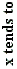# Graph of a Polynomial Defined by Its Roots

Most of the numbers - roots, the degree of the polynomial, the minimum and maximum bounds on both x- and y-axis - are clickable. To change a value up click (or drag the cursor to speed things up) a little to the right of the vertical center line of a number. Clicking on the left has the opposite effect.

### This applet requires Sun's Java VM 2 which your browser may perceive as a popup. Which it is not. If you want to see the applet work, visit Sun's website at https://www.java.com/en/download/index.jsp, download and install Java VM and enjoy the applet.

 What if applet does not run?

As in the companion program that defines polynomials by their coefficients, this one, too, allows one to make a few general observations on the behavior of polynomials depending on the degree and distribution of their roots

1. Polynomials are unbounded functions. At both ends of the x-axis, unless they are constant, polynomials grow without bound in their absolute value. Sometimes they go to the positive infinity and sometimes to the negative infinity. What exactly happens depends on three factors: the parity of their degree, the sign of their main coefficient, and the side of the x-axis we are talking about. The following table summarizes all possible behaviors:

 Degree's Parity Odd Even+ ∞ + ∞ - ∞ + ∞

provided the main coefficient is positive. For a negative coefficient, the signs in the table are reversed.

2. Polynomials may or may not have repeated roots. The behavior of a polynomial in a vicinity of a root depends on the parity of the root's multiplicity. If the multiplicity is even, the graph at this point is tangent to and stays on one side of the x-axis. Otherwise, the two cross and the graph is of different signs on the two sides of the root. If the multiplicity of a root is great than one, the graph is tangent to the x-axis regardless of the root's parity.

3. Changing the value of the main coefficient stretches or squeezes the graph vertically. Changing the coefficient's sign flips the graph around the x-axis.• What Is Line?
• Functions, what are they?
• Cartesian Coordinate System
• Addition and Subtraction of Functions
• Function, Derivative and Integral
• Graph of a Polynomial of arbitrary degree
• Inflection Points of Fourth Degree Polynomials
• Lagrange Interpolation (an Interactive Gizmo)
• Equations of a Straight Line
• Taylor Series Approximation to Cosine
• Taylor Series Approximation to Cosine
• Linear Function with Coefficients in Arithmetic Progression
• Sine And Cosine Are Continuous Functions
•